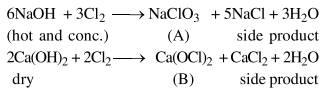# Solve this followingQuestion:

In the following reactions products $(A)$ and (B), respectively, are :

$\mathrm{NaOH}+\mathrm{Cl}_{2} \rightarrow(\mathrm{A})+$ side products

(hot and conc.)

$\mathrm{Ca}(\mathrm{OH})_{2}+\mathrm{Cl}_{2} \rightarrow(\mathrm{B})+$ side products

(dry)

1. $\mathrm{NaClO}_{3}$ and $\mathrm{Ca}(\mathrm{OCl})_{2}$

2. $\mathrm{NaOCl}$ and $\mathrm{Ca}\left(\mathrm{ClO}_{3}\right)_{2}$

3. $\mathrm{NaClO}_{3}$ and $\mathrm{Ca}\left(\mathrm{ClO}_{3}\right)_{2}$

4. $\mathrm{NaOCl}$ and $\mathrm{Ca}(\mathrm{OCl})_{2}$

Correct Option: 1

Solution: